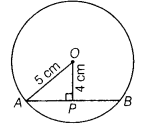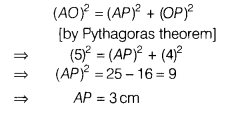# Find the length of a chord which is at a distance of 4 cm from the centre of a circle of radius 5 cm

Find the length of a chord which is at a distance of 4 cm from the centre of a circle of radius 5 cm.

Given, the perpendicular distance,OP = 4cm
and radius of circle, OA = 5 cm
In right angled ∆APO,We know that, the perpendicular drawn from the centre of a circle to a chord divides the chord into two equal parts.